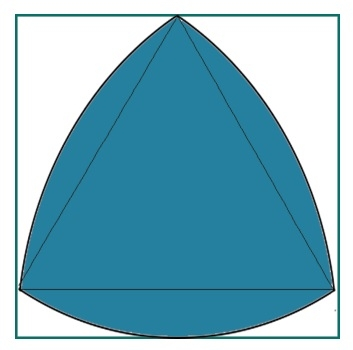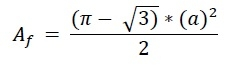# Biggest Reuleaux Triangle within A Square?

Here we will see the area of biggest Reuleaux triangle inscribed within a square. The side of the square is ‘a’. And the height of the Reuleaux triangle is h.The height of the Reuleaux triangle is same as a. So a = h. So the area of Reuleaux triangle is −## Example

#include <iostream>
#include <cmath>
using namespace std;
float areaReuleaux(float a) { //side of square is a
if (a < 0) //if a is negative it is invalid
return -1;
float area = ((3.1415 - sqrt(3)) * (a) * (a))/2;
return area;
}
int main() {
float side = 8;
cout << "Area of Reuleaux Triangle: " << areaReuleaux(side);
}

## Output

Area of Reuleaux Triangle: 45.1024